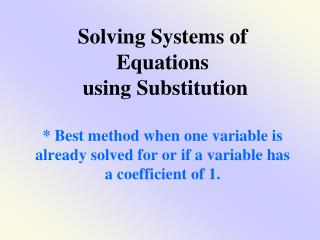DownloadDownload PresentationSolving Systems of Equations using Substitution

# Solving Systems of Equations using Substitution

Télécharger la présentation## Solving Systems of Equations using Substitution

- - - - - - - - - - - - - - - - - - - - - - - - - - - E N D - - - - - - - - - - - - - - - - - - - - - - - - - - -
##### Presentation Transcript

1. Solving Systems of Equations using Substitution* Best method when one variable is already solved for or if a variable has a coefficient of 1.

2. Solving Systems of Equations using Substitution Steps: 1. Solve One equation for One variable.( y= ; x= ; a=) 2. Substitute equation from step one into other equation. (Get an equation with only one variable.) 3. Solve for the variable in step 2. 4. Go back and use the found variable in step 3 to find second variable. 5. Check the solution in both equations of the system. WHAT DO WE DO???? CLICK TO SEE AN EXAMPLE

3. Ex. 1: Solve the system of equations. y= 4x 3x+y=-21 STEP1: y=4x (already solved for y) STEP 2: Substitute for y in the second equation 3x + y = -21 and it becomes: 3x +4x =-21

4. GIVEN EXAMPLE: y= 4x 3x+y=-21 STEP 3: Solve for the variable. 3x + 4x=-21 7x=-21 x=-3

5. GIVEN EXAMPLE: y= 4x 3x+y=-21 STEP 4: Solve for the other variable. Use x=-3 and y=4x. y=4x and x = -3 therefore: y=4(-3) or y = -12 Solution to the system is (-3,-12).

6. GIVEN EXAMPLE: y= 4x 3x+y=-21 Check solution. ( -3,-12) y=4x -12=4(-3) -12=-12 3x+y=-21 3(-3)+(-12)=-21 -9+(-12)=-21 -21=-21

7. Solving Systems of Equations using Substitution Steps: 1. Solve One equation for One variable.( y= ; x= ; a=) 2. Substitute equation from step one into other equation. (Get an equation with only one variable.) 3. Solve for the first variable. 4. Go back and use the found variable in step 3 to find second variable. 5. Check the solution in both equations of the system. QUESTIONS?

8. GIVEN EXAMPLE: x + y=10 5x - y=2 STEP1: Solve for y. x + y = 10 y = -x +10 STEP 2: Substitute for y in the second equation 5x - y= 2 and it becomes: 5x - (-x+10) = 2

9. GIVEN EXAMPLE: x + y=10 5x - y=2 STEP 3: Solve for the variable. 5x-(-x+10)=2 5x+x-10=2 6x-10=2 6x=12 x=2

10. GIVEN EXAMPLE: x + y=10 5x - y=2 STEP 4: Solve for the other variable. Use x=2 and x+y=10. x=2 and x+y = 10 therefore: 2+y=10 and y = 8 Solution to the system is (2,8).

11. GIVEN EXAMPLE: x + y=10 5x - y=2 Check solution. (2,8) 5x-y=2 5(2)-(8)=2 10-8=2 2=2 x + y=10 2+8=10 10=10